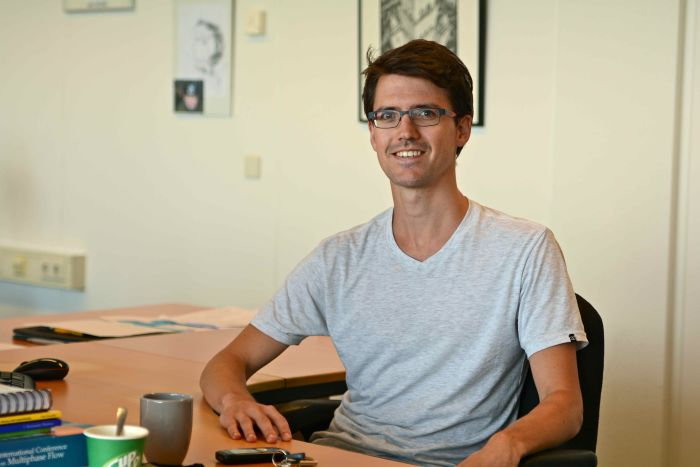# Mathematical Consultancy

Some problems can be solved best by applying mathematics. We help you formulate your issue in mathematical terms and then solve it.

## Mathematical problem solvers

Many practical problems can be solved with mathematics. First, the problem must be formulated in mathematical terms. Then it can be analyzed and solved. Mathematical consultancy can contribute to each of these phases. Most of VORtech's mathematical consultants have a PhD in Applied Mathematics (or a related field) and many years of experience.### Describing the problem in mathematical terms…

Once a problem is described in mathematical terms, the entire mathematical toolkit becomes available to find a solution. VORtech managed to find solutions to various nagging problems:

• We have looked into a production process for artificial fibers, which used to fail often. A mathematical description of the problem had already been made, but the company R&D department couldn’t reach any useful results from it. VORtech improved the mathematical description and found the cause of the failures in the production process. Once that insight was available, the process could be re-engineered. It now runs reliably.
• A producer of tents wanted to have a design for tents without unnecessary folds, as folds mean that more material is used than would strictly be needed. Also, it doesn’t look nice. VORtech developed an algorithm to minimize unnecessary folds.

### … and solving it

Sometimes, experts in R&D departments lack mathematical knowledge. They may have the mathematical formulation of their problem, but they lack the knowledge about methods to solve it. In that case, our consultants can be of help as well. A few examples:

• One of our customers is an asset-management company that needs to decide periodically about their infrastructure investments. They already had an optimization method to make an optimal choice from all the investment proposals, but that method could only optimize a period of one year. Being able to optimize across multiple years was interesting for this customer to allow them to exploit developments for instance in materials prices. VORtech extended the existing optimization method to cover multiple years. This work was done in close collaboration with asset-management experts.
• Experts at an environmental research institute use an optimization method to determine the optimal combination of policy decisions to reach a certain environmental target. The method that they used tended to give very different solutions when the input was changed only a little. VORtech analyzed the optimization problem and the solution method and found an explanation for this behavior. By adapting the optimization method, the results could be made far more consistent.